CoCalc Shared FilesCDS-102 / Lab Week 08 - Statistical distribution of speed-of-light measurements / CDS-102 Lab Week 08 Workbook.html
Author: James Glasbrenner
Views : 10
Description: Jupyter html version of CDS-102/Lab Week 08 - Statistical distribution of speed-of-light measurements/CDS-102 Lab Week 08 Workbook.ipynb
CDS-102 Lab Week 08 Workbook

# CDS-102: Lab 8 Workbook¶

## Helena Gray¶

### March 23, 2017¶

The code below imports the tidyverse package which will be used for analysis. It then reads in a comma separate value file into a variable named 'newcomb'.

In :
# Run this code block to load the Tidyverse package
.libPaths(new = "~/Rlibs")
library(tidyverse)
newcomb

Loading tidyverse: ggplot2
Conflicts with tidy packages ---------------------------------------------------
filter(): dplyr, stats
lag():    dplyr, stats

Xx
1 28
2 -44
3 29
4 30
5 24
6 28
7 37
8 32
9 36
10 27
11 26
12 28
13 29
14 26
15 27
16 22
17 23
18 20
19 25
20 25
21 36
22 23
23 31
24 32
25 24
26 27
27 33
28 16
29 24
30 29
3732
3825
3928
4024
4140
4221
4331
4432
4528
4626
4730
4827
4926
5024
5132
5229
5334
54-2
5525
5619
5736
5829
5930
6022
6128
6233
6339
6425
6516
6623

The code below plots a histogram of the dataset using ggplot2.

In :
newcomb.ggplot<-ggplot(data=newcomb)  + geom_histogram(binwidth = 1, mapping = aes(x=x), alpha=.5)
ggsave("newcomb.ggplot.png", plot = newcomb.ggplot, device="png", scale=1, width=5, height=4)
newcomb.ggplot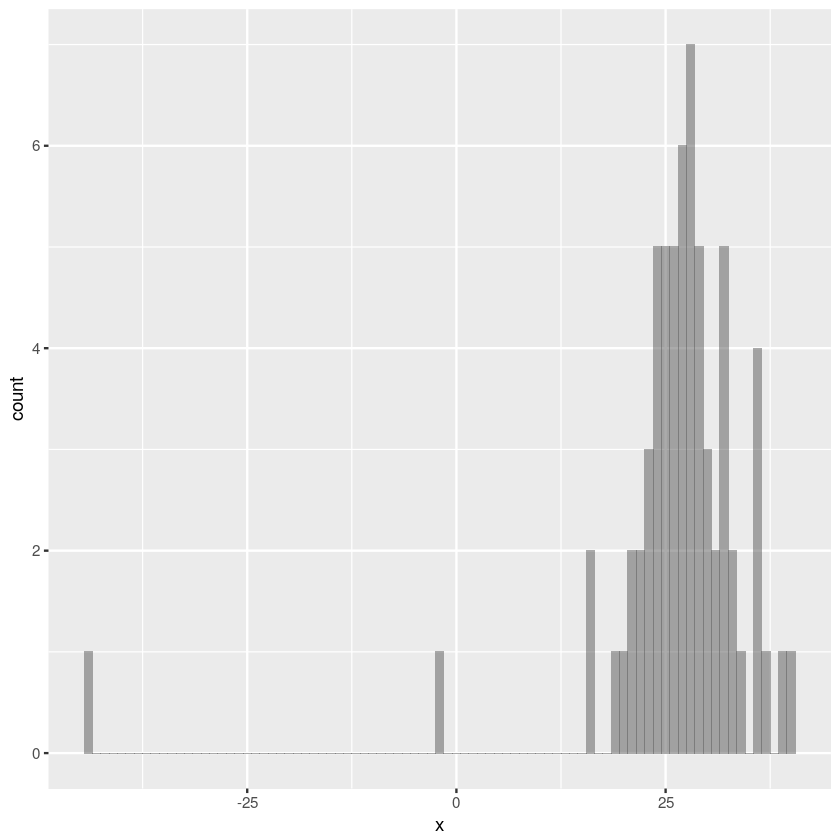A variable is created called 'newcomb_1' which filters out all the values of 'x' under 0.

In :
newcomb_1<-filter(newcomb, x>0)


The code below gives summary statistics for the filtered data set 'newcomb' and the unfiltered dataset 'newcomb_1'.

In :
stat.table.filtered<-summarise(newcomb_1,
mean=mean(x), max=max(x),min=min(x),range=max(x)-min(x),var=var(x), sd=sd(x))
stat.table.filtered

stat.table<-summarise(newcomb,
mean=mean(x), max=max(x),min=min(x),range=max(x)-min(x),var=var(x), sd=sd(x))
stat.table

meanmaxminrangevarsd
27.75 40 16 24 25.841275.083431
meanmaxminrangevarsd
26.2121240 -44 84 115.462 10.74532

The code below uses the dnorm() function to calculate the probability distribution for the filtered and unfiltered datasets. It takes the 'x' values (from the distribution) as input and returns the probability (taken from the bell curve) as output.

In :
newcomb_1_pdf<-dnorm(x=newcomb_1$x , mean = 27.75 , sd = 5.083431) newcomb_pdf<-dnorm(x=newcomb$x , mean = 26.21212 , sd = 10.745)


The code below converts the histogram (unfiltered dataset only) into a probability mass function using the ggplot_build() function and stores the PMF values in a tibble.

In :
data.ggplot.full <- ggplot_build(newcomb.ggplot)
data.ggplot.table <- data.ggplot.full$data[] histogram.table <- tibble(x = data.ggplot.table$x, density = data.ggplot.table$y, frequency = data.ggplot.table$count)
#data.ggplot.table


A plot is created that overlays the normal distribution models on top of the PMF to see how well that they agree.

In :
options(repr.plot.width = 6, repr.plot.height = 4)
data.ggplot_1<-ggplot(data=histogram.table)  + geom_col(mapping = aes(x=x, y=density), alpha=.5) + stat_function(fun=dnorm, args=list(mean=mean(newcomb$x),sd=sd(newcomb$x)), color= "red")
ggsave("data.ggplot_1.png", plot = data.ggplot_1, device="png", scale=1, width=5, height=4)
data.ggplot_1

options(repr.plot.width = 6, repr.plot.height = 4)
data.ggplot_2<-ggplot(data=histogram.table)  + geom_col(mapping = aes(x=x, y=density), alpha=.5) + stat_function(fun=dnorm, args=list(mean=mean(newcomb_1$x),sd=sd(newcomb_1$x)), color= "red")
ggsave("data.ggplot_2.png", plot = data.ggplot_2, device="png", scale=1, width=5, height=4)
data.ggplot_2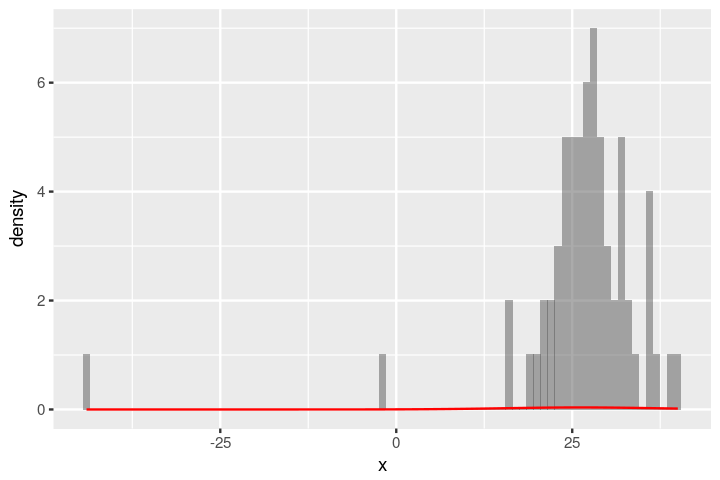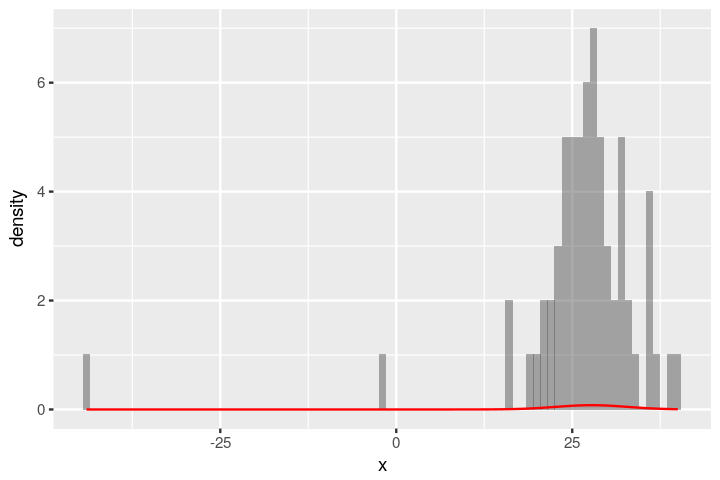The code below creates a cumulative distribution function generator for both the unfiltered and filtered datasets using the ecdf() function. Two tibbles are created, each with two columns. In each tibble the first column is t that contains values running from -45 to 45 in increments of 0.1, and the second column is cdf containing values of the cdf generator evaluated at the same values of t.

In :
newcomb_1_range_vector <- seq(-45,45.1)
newcomb_1_cdf_generator <- ecdf(newcomb_1$x) newcomb_1_cdf <- tibble(score = newcomb_1_range_vector, CDF = newcomb_1_cdf_generator(newcomb_1_range_vector)) newcomb_range_vector <- seq(-45,45.1) newcomb_cdf_generator <- ecdf(newcomb$x)
newcomb_cdf <- tibble(score = newcomb_range_vector, CDF = newcomb_cdf_generator(newcomb_range_vector))


The code below creates the corresponding CDF for the normal distribution models (both unfiltered and filtered) using the qnorm() function. The two CDFs are evaluated in the range from 0 to 1 in increments of 0.01. The results are stored in two tibbles, each with two columns (this includes t, which is just the 0 to 1 range in increments of 0.01, and the CDF values).

In :
newcomb_1_percentiles <- seq(0, 1, 0.01)
newcomb_1_cdf_model <- tibble(CDF = newcomb_1_percentiles,
score = qnorm(p = newcomb_1_percentiles, mean = 27.75, sd = 5.08))

newcomb_percentiles <- seq(0, 1, 0.01)
newcomb_cdf_model <- tibble(CDF = newcomb_percentiles,
score = qnorm(p = newcomb_percentiles, mean = 26.21, sd = 10.74))


The code below visually compares the CDFs of models and datasets to estimate the quality of agreement. 2 plots are created that overlay the two model CDFs on top of the unfiltered and filtered datasets CDFs.

In :
# Create Plot
ggplot(newcomb_1_cdf) +
geom_line(mapping = aes(x = score, y = CDF), color = "cyan4", size = 2) +
geom_line(data = newcomb_1_cdf_model, mapping = aes(x = score, y = CDF), color = "black", size = 1)

# Create Plot
ggplot(newcomb_cdf) +
geom_line(mapping = aes(x = score, y = CDF), color = "lightcoral", size = 2) +
geom_line(data = newcomb_cdf_model, mapping = aes(x = score, y = CDF), color = "black", size = 1)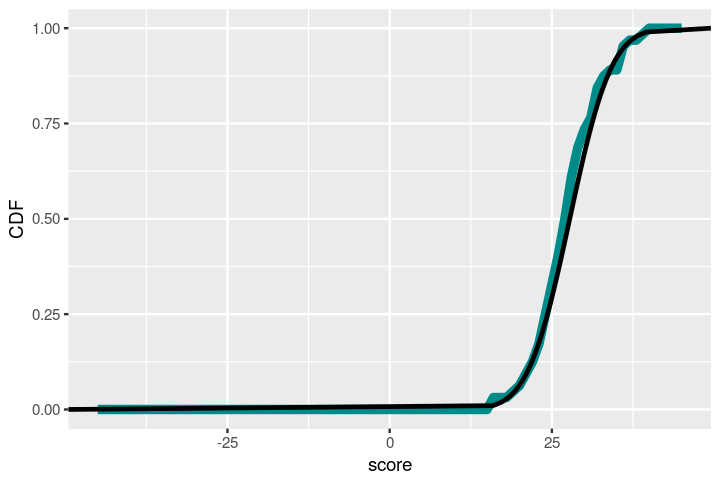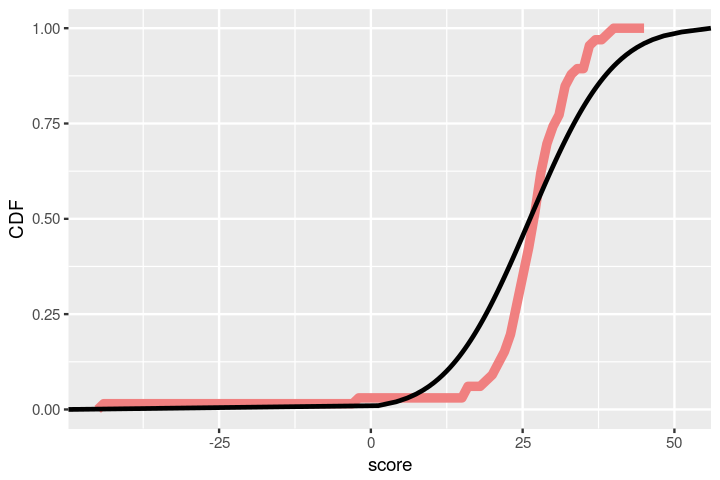The code below calculates the confidence interval for the unfiltered and filtered datasets, and then prints out the two versions of the experimental result in the format mean ± confidence interval.

In :
ci_filtered<- 2 * stat.table.filtered$sd ci<- 2 * stat.table$sd
cat("The confidence interval for the unfiltered dataset is ", stat.table$mean, "+-",ci,"\n") cat("The confidence interval for the unfiltered dataset is ", stat.table.filtered$mean, "+-",ci_filtered)

The confidence interval for the unfiltered dataset is  26.21212 +- 21.49065
The confidence interval for the unfiltered dataset is  27.75 +- 10.16686

The code below performs a 1 sample t-test which can tell us the probability that the confidence interval obtained contains the true mean.

In :
t.test(x = newcomb$x, mu = 33.02)   One Sample t-test data: newcomb$x
t = -5.1471, df = 65, p-value = 2.648e-06
alternative hypothesis: true mean is not equal to 33.02
95 percent confidence interval:
23.57059 28.85365
sample estimates:
mean of x
26.21212

In [ ]: